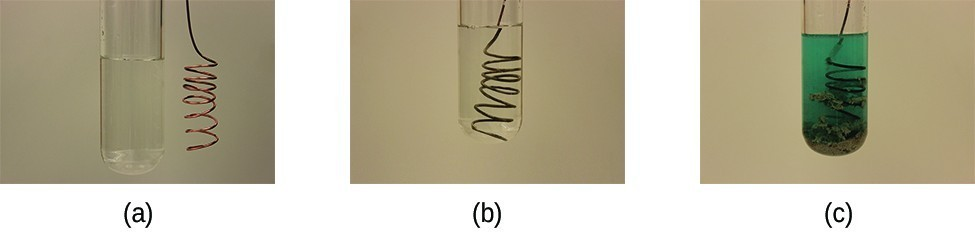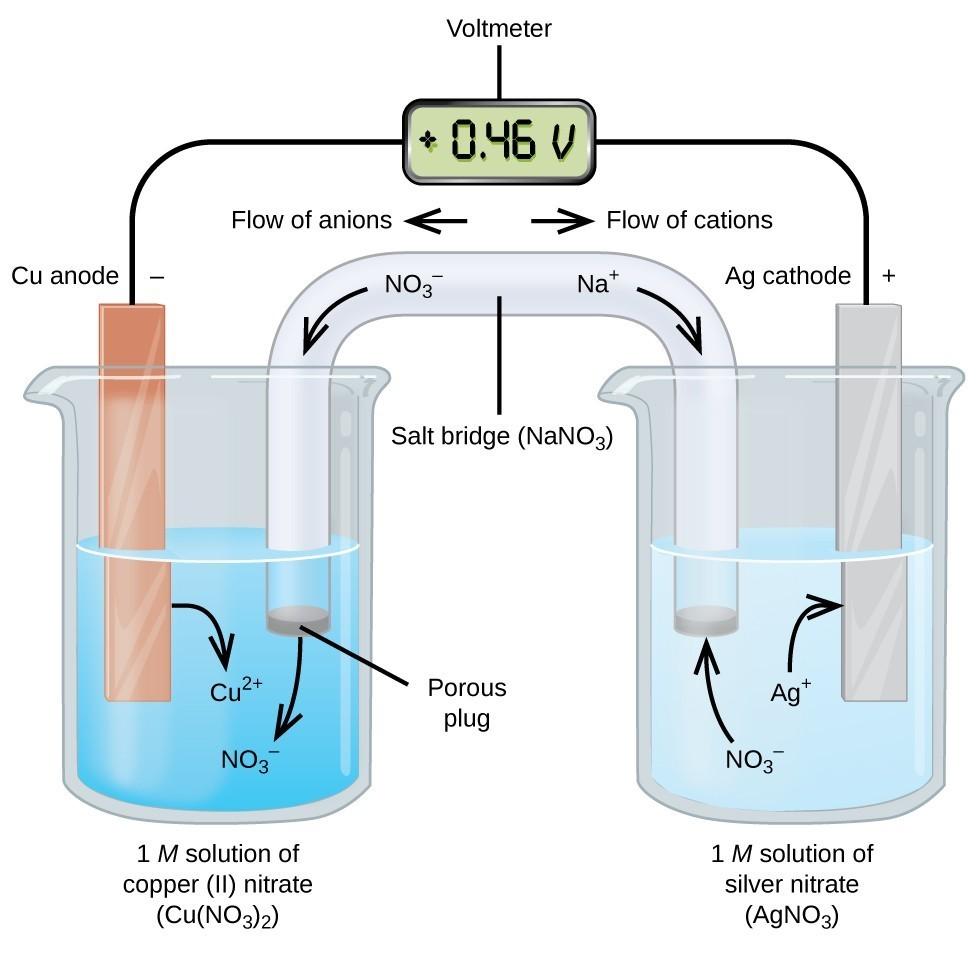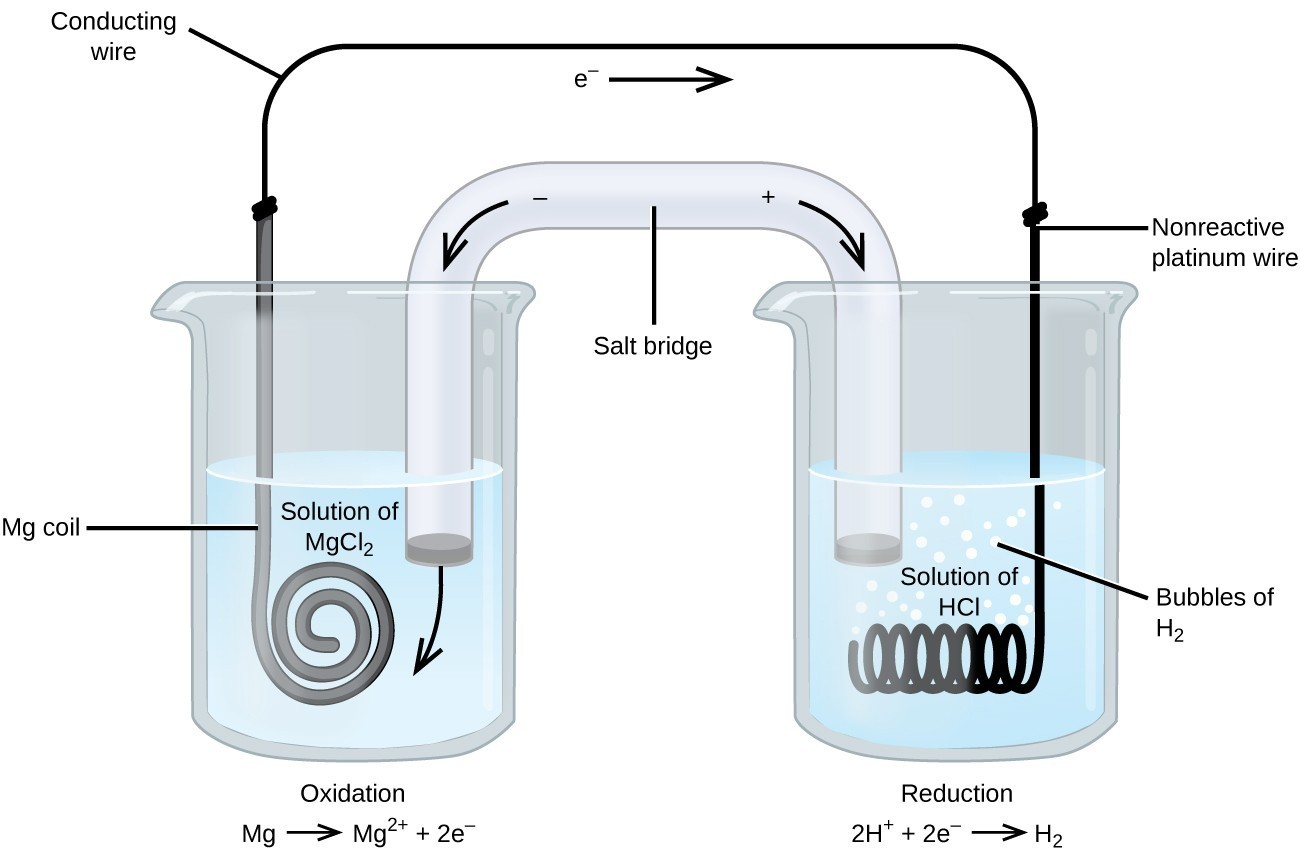Galvanic Cells

Learning Objectives

By the end of this module, you will be able to:

• Use cell notation to describe galvanic cells
• Describe the basic components of galvanic cells

Galvanic cells, also known as voltaic cells, are electrochemical cells in which spontaneous oxidation-reduction reactions produce electrical energy. In writing the equations, it is often convenient to separate the oxidation-reduction reactions into half-reactions to facilitate balancing the overall equation and to emphasize the actual chemical transformations.

Consider what happens when a clean piece of copper metal is placed in a solution of silver nitrate (Figure 1). As soon as the copper metal is added, silver metal begins to form and copper ions pass into the solution. The blue color of the solution on the far right indicates the presence of copper ions. The reaction may be split into its two half-reactions. Half-reactions separate the oxidation from the reduction, so each can be considered individually.

$\begin{array}{rl}{}\text{oxidation:}&\text{Cu}\left(s\right)\longrightarrow {\text{Cu}}^{2+}\left(aq\right)+{\text{2e}}^{-}\\ \text{reduction:}&\text{2}\times \left({\text{Ag}}^{\text{+}}\left(aq\right)+{\text{e}}^{-}\longrightarrow \text{Ag}\left(s\right)\right)\text{ or }2{\text{Ag}}^{\text{+}}\left(aq\right)+{\text{2e}}^{-}\longrightarrow \text{2Ag}\left(s\right)\\ \\ \text{overall:}&2{\text{Ag}}^{\text{+}}\left(aq\right)+\text{Cu}\left(s\right)\longrightarrow \text{2Ag}\left(s\right)+{\text{Cu}}^{2+}\left(aq\right)\end{array}$

The equation for the reduction half-reaction had to be doubled so the number electrons “gained” in the reduction half-reaction equaled the number of electrons “lost” in the oxidation half-reaction.Figure 1. When a clean piece of copper metal is placed into a clear solution of silver nitrate (a), an oxidation-reduction reaction occurs that results in the exchange of Cu2+ for Ag+ ions in solution. As the reaction proceeds (b), the solution turns blue (c) because of the copper ions present, and silver metal is deposited on the copper strip as the silver ions are removed from solution. (credit: modification of work by Mark Ott)

Galvanic or voltaic cells involve spontaneous electrochemical reactions in which the half-reactions are separated (Figure 2) so that current can flow through an external wire. The beaker on the left side of the figure is called a half-cell, and contains a 1 M solution of copper(II) nitrate [Cu(NO3)2] with a piece of copper metal partially submerged in the solution. The copper metal is an electrode. The copper is undergoing oxidation; therefore, the copper electrode is the anode. The anode is connected to a voltmeter with a wire and the other terminal of the voltmeter is connected to a silver electrode by a wire. The silver is undergoing reduction; therefore, the silver electrode is the cathode.

The half-cell on the right side of the figure consists of the silver electrode in a 1 M solution of silver nitrate (AgNO3). At this point, no current flows—that is, no significant movement of electrons through the wire occurs because the circuit is open. The circuit is closed using a salt bridge, which transmits the current with moving ions. The salt bridge consists of a concentrated, nonreactive, electrolyte solution such as the sodium nitrate (NaNO3) solution used in this example. As electrons flow from left to right through the electrode and wire, nitrate ions (anions) pass through the porous plug on the left into the copper(II) nitrate solution. This keeps the beaker on the left electrically neutral by neutralizing the charge on the copper(II) ions that are produced in the solution as the copper metal is oxidized. At the same time, the nitrate ions are moving to the left, sodium ions (cations) move to the right, through the porous plug, and into the silver nitrate solution on the right. These added cations “replace” the silver ions that are removed from the solution as they were reduced to silver metal, keeping the beaker on the right electrically neutral. Without the salt bridge, the compartments would not remain electrically neutral and no significant current would flow. However, if the two compartments are in direct contact, a salt bridge is not necessary. The instant the circuit is completed, the voltmeter reads +0.46 V, this is called the cell potential. The cell potential is created when the two dissimilar metals are connected, and is a measure of the energy per unit charge available from the oxidation-reduction reaction. The volt is the derived SI unit for electrical potential:

$\text{volt}=V=\frac{{\text{kg/m}}^{2}}{{\text{A/s}}^{3}}=\frac{\text{J}}{\text{A/s}}=\frac{\text{J}}{\text{C}}$

In this equation, A is the current in amperes and C the charge in coulombs. Note that volts must be multiplied by the charge in coulombs (C) to obtain the energy in joules (J).Figure 2. In this standard galvanic cell, the half-cells are separated; electrons can flow through an external wire and become available to do electrical work.

When the electrochemical cell is constructed in this fashion, a positive cell potential indicates a spontaneous reaction and that the electrons are flowing from the left to the right. There is a lot going on in Figure 2, so it is useful to summarize things for this system:

• Electrons flow from the anode to the cathode: left to right in the standard galvanic cell in the figure.
• The electrode in the left half-cell is the anode because oxidation occurs here. The name refers to the flow of anions in the salt bridge toward it.
• The electrode in the right half-cell is the cathode because reduction occurs here. The name refers to the flow of cations in the salt bridge toward it.
• Oxidation occurs at the anode (the left half-cell in the figure).
• Reduction occurs at the cathode (the right half-cell in the figure).
• The cell potential, +0.46 V, in this case, results from the inherent differences in the nature of the materials used to make the two half-cells.
• The salt bridge must be present to close (complete) the circuit and both an oxidation and reduction must occur for current to flow.

There are many possible galvanic cells, so a shorthand notation is usually used to describe them. The cell notation (sometimes called a cell diagram) provides information about the various species involved in the reaction. This notation also works for other types of cells. A vertical line, |, denotes a phase boundary and a double line, ‖, the salt bridge. Information about the anode is written to the left, followed by the anode solution, then the salt bridge (when present), then the cathode solution, and, finally, information about the cathode to the right. The cell notation for the galvanic cell in Figure 2 is then

$\text{Cu}\left(s\right)\mid {\text{Cu}}^{2+}\left(aq\text{, 1}M\right)\parallel {\text{Ag}}^{\text{+}}\left(aq\text{, 1}M\right)\mid \text{Ag}\left(s\right)$

Note that spectator ions are not included and that the simplest form of each half-reaction was used. When known, the initial concentrations of the various ions are usually included.

One of the simplest cells is the Daniell cell. It is possible to construct this battery by placing a copper electrode at the bottom of a jar and covering the metal with a copper sulfate solution. A zinc sulfate solution is floated on top of the copper sulfate solution; then a zinc electrode is placed in the zinc sulfate solution. Connecting the copper electrode to the zinc electrode allows an electric current to flow. This is an example of a cell without a salt bridge, and ions may flow across the interface between the two solutions.

Some oxidation-reduction reactions involve species that are poor conductors of electricity, and so an electrode is used that does not participate in the reactions. Frequently, the electrode is platinum, gold, or graphite, all of which are inert to many chemical reactions. One such system is shown in Figure 3. Magnesium undergoes oxidation at the anode on the left in the figure and hydrogen ions undergo reduction at the cathode on the right. The reaction may be summarized as

$\begin{array}{rl}\text{oxidation:}&\text{Mg}\left(s\right)\longrightarrow\text{Mg}^{2+}\left(aq\right)+\text{2e}^{-}\\ \text{reduction:}&{\text{2H}}^{\text{+}}\left(aq\right)+{\text{2e}}^{-}\longrightarrow {\text{H}}_{2}\left(g\right) \\ \\ \text{overall:}&\text{Mg}\left(s\right)+{\text{2H}}^{\text{+}}\left(aq\right)\longrightarrow {\text{Mg}}^{2+}\left(aq\right)+{\text{H}}_{2}\left(g\right)\end{array}$

The cell used an inert platinum wire for the cathode, so the cell notation is

$\text{Mg}\left(s\right)\mid {\text{Mg}}^{2+}\left(aq\right)\parallel {\text{H}}^{\text{+}}\left(aq\right)\mid {\text{H}}_{2}\left(g\right)\mid \text{Pt}\left(s\right)$

The magnesium electrode is an active electrode because it participates in the oxidation-reduction reaction. Inert electrodes, like the platinum electrode in Figure 3, do not participate in the oxidation-reduction reaction and are present so that current can flow through the cell. Platinum or gold generally make good inert electrodes because they are chemically unreactive.

Example 1: Using Cell Notation

Question 1

Consider a galvanic cell consisting of $\text{2Cr}\left(s\right)+{\text{3Cu}}^{2+}\left(aq\right)\longrightarrow {\text{2Cr}}^{3+}\left(aq\right)+\text{3Cu}\left(s\right)$

Write the oxidation and reduction half-reactions and write the reaction using cell notation. Which reaction occurs at the anode? The cathode?

Question 2

Consider a galvanic cell consisting of $5{\text{Fe}}^{2+}\left(aq\right)+{\text{MnO}}_{4}{}^{-}\left(aq\right)+{\text{8H}}^{\text{+}}\left(aq\right)\longrightarrow {\text{5Fe}}^{3+}\left(aq\right)+{\text{Mn}}^{2+}\left(aq\right)+{\text{4H}}_{2}\text{O}\left(l\right)$

Write the oxidation and reduction half-reactions and write the reaction using cell notation. Which reaction occurs at the anode? The cathode?

Use cell notation to describe the galvanic cell where copper(II) ions are reduced to copper metal and zinc metal is oxidized to zinc ions.Figure 3. The oxidation of magnesium to magnesium ion occurs in the beaker on the left side in this apparatus; the reduction of hydrogen ions to hydrogen occurs in the beaker on the right. A nonreactive, or inert, platinum wire allows electrons from the left beaker to move into the right beaker. The overall reaction is: Mg + 2H+ ⟶ Mg2+ + H2, which is represented in cell notation as: Mg(s)│Mg2+(aq)║H+(aq)│H2(g)│Pt(s).

Key Concepts and Summary

Electrochemical cells typically consist of two half-cells. The half-cells separate the oxidation half-reaction from the reduction half-reaction and make it possible for current to flow through an external wire. One half-cell, normally depicted on the left side in a figure, contains the anode. Oxidation occurs at the anode. The anode is connected to the cathode in the other half-cell, often shown on the right side in a figure. Reduction occurs at the cathode. Adding a salt bridge completes the circuit allowing current to flow. Anions in the salt bridge flow toward the anode and cations in the salt bridge flow toward the cathode. The movement of these ions completes the circuit and keeps each half-cell electrically neutral. Electrochemical cells can be described using cell notation. In this notation, information about the reaction at the anode appears on the left and information about the reaction at the cathode on the right. The salt bridge is represented by a double line, ‖. The solid, liquid, or aqueous phases within a half-cell are separated by a single line, |. The phase and concentration of the various species is included after the species name. Electrodes that participate in the oxidation-reduction reaction are called active electrodes. Electrodes that do not participate in the oxidation-reduction reaction but are there to allow current to flow are inert electrodes. Inert electrodes are often made from platinum or gold, which are unchanged by many chemical reactions.

Exercises

1. Write the following balanced reactions using cell notation. Use platinum as an inert electrode, if needed.
1. $\text{Mg}\left(s\right)+{\text{Ni}}^{2+}\left(aq\right)\longrightarrow {\text{Mg}}^{2+}\left(aq\right)+\text{Ni}\left(s\right)$
2. $2{\text{Ag}}^{\text{+}}\left(aq\right)+\text{Cu}\left(s\right)\longrightarrow {\text{Cu}}^{2+}\left(aq\right)+\text{2Ag}\left(s\right)$
3. $\text{Mn}\left(s\right)+\text{Sn}\left(\text{NO}_{3}\right)_{2}\left(aq\right)\longrightarrow \text{Mn}\left(\text{NO}_{3}\right)_{2}\left(aq\right)+\text{Au}\left(s\right)$
4. $3\text{CuNO}_{3}\left(aq\right)+\text{Au}\left(\text{NO}_{3}\right)_{3}\left(aq\right)\longrightarrow3\text{Cu}\left(\text{NO}_{3}\right)_{2}\left(aq\right)+\text{Au}\left(s\right)$
2. Given the following cell notations, determine the species oxidized, species reduced, and the oxidizing agent and reducing agent, without writing the balanced reactions.
1. $\text{Mg}\left(s\right)\mid {\text{Mg}}^{2+}\left(aq\right)\parallel {\text{Cu}}^{2+}\left(aq\right)\mid \text{Cu}\left(s\right)$
2. $\text{Ni}\left(s\right)\mid {\text{Ni}}^{2+}\left(aq\right)\parallel {\text{Ag}}^{\text{+}}\left(aq\right)\mid \text{Ag}\left(s\right)$
3. For the cell notations in the previous problem, write the corresponding balanced reactions.
4. Balance the following reactions and write the reactions using cell notation. Ignore any inert electrodes, as they are never part of the half-reactions.
1. $\text{Al}\left(s\right)+{\text{Zr}}^{4+}\left(aq\right)\longrightarrow {\text{Al}}^{3+}\left(aq\right)+\text{Zr}\left(s\right)$
2. ${\text{Ag}}^{\text{+}}\left(aq\right)+\text{NO}\left(g\right)\longrightarrow \text{Ag}\left(s\right)+{\text{NO}}_{3}{}^{-}\left(aq\right)\left(\text{acidic solution}\right)$
3. ${\text{SiO}}_{3}{}^{2-}\left(aq\right)+\text{Mg}\left(s\right)\longrightarrow \text{Si}\left(s\right)+\text{Mg}{\left(\text{OH}\right)}_{2}\left(s\right)\text{(basic solution)}$
4. ${\text{ClO}}_{3}{}^{-}\left(aq\right)+{\text{MnO}}_{2}\left(s\right)\longrightarrow {\text{Cl}}^{-}\left(aq\right)+{\text{MnO}}_{4}{}^{-}\left(aq\right)\text{(basic solution)}$
5. Identify the species oxidized, species reduced, and the oxidizing agent and reducing agent for all the reactions in the previous problem.
6. From the information provided, use cell notation to describe the following systems:
1. In one half-cell, a solution of Pt(NO3)2 forms Pt metal, while in the other half-cell, Cu metal goes into a Cu(NO3)2 solution with all solute concentrations 1 M.
2. The cathode consists of a gold electrode in a 0.55 M Au(NO3)3 solution and the anode is a magnesium electrode in 0.75 M Mg(NO3)2 solution.
3. One half-cell consists of a silver electrode in a 1 M AgNO3 solution, and in the other half-cell, a copper electrode in 1 M Cu(NO3)2 is oxidized.
7. Why is a salt bridge necessary in galvanic cells like the one in Figure 2?
8. An active (metal) electrode was found to gain mass as the oxidation-reduction reaction was allowed to proceed. Was the electrode part of the anode or cathode? Explain.
9. An active (metal) electrode was found to lose mass as the oxidation-reduction reaction was allowed to proceed. Was the electrode part of the anode or cathode? Explain.
10. The mass of three different metal electrodes, each from a different galvanic cell, were determined before and after the current generated by the oxidation-reduction reaction in each cell was allowed to flow for a few minutes. The first metal electrode, given the label A, was found to have increased in mass; the second metal electrode, given the label B, did not change in mass; and the third metal electrode, given the label C, was found to have lost mass. Make an educated guess as to which electrodes were active and which were inert electrodes, and which were anode(s) and which were the cathode(s).

Glossary

active electrode: electrode that participates in the oxidation-reduction reaction of an electrochemical cell; the mass of an active electrode changes during the oxidation-reduction reaction

anode: electrode in an electrochemical cell at which oxidation occurs; information about the anode is recorded on the left side of the salt bridge in cell notation

cathode: electrode in an electrochemical cell at which reduction occurs; information about the cathode is recorded on the right side of the salt bridge in cell notation

cell notation: shorthand way to represent the reactions in an electrochemical cell

cell potential: difference in electrical potential that arises when dissimilar metals are connected; the driving force for the flow of charge (current) in oxidation-reduction reactions

galvanic cell: electrochemical cell that involves a spontaneous oxidation-reduction reaction; electrochemical cells with positive cell potentials; also called a voltaic cell

inert electrode: electrode that allows current to flow, but that does not otherwise participate in the oxidation-reduction reaction in an electrochemical cell; the mass of an inert electrode does not change during the oxidation-reduction reaction; inert electrodes are often made of platinum or gold because these metals are chemically unreactive.

voltaic cell: another name for a galvanic cell